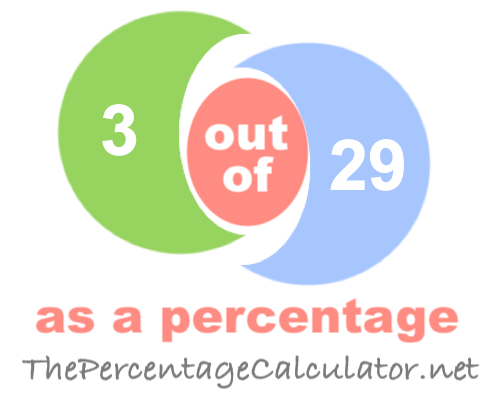What is 3 out of 29 as a percentage?When you ask "What is 3 out of 29?" you want to know what percent 3 is out of 29.

Here are step-by-step instructions showing you how we calculated 3 out of 29 as a percentage:

The first step is to divide 3 by 29 to get the answer in decimal form:

3 ÷ 29 ≈ 0.1034

Then, we multiplied the answer from the first step by one hundred to get the answer as a percentage:

0.1034 × 100 = 10.34%

We can prove that the answer is correct by taking 10.34 percent of 29 to get 3:

(29 × 10.34) ÷ 100 ≈ 3

Note that our calculator rounds the answers up to two decimals if necessary. Once again, the answer is as follows:

≈ 10.34%

Out of as a Percentage Calculator
To solve another problem, please submit it below:

out of

What is 3 out of 30 as a percentage?
The solution to "What is 3 out of 29 as a percentage?" is not the only answer we have. Go here for the next solution on our list.